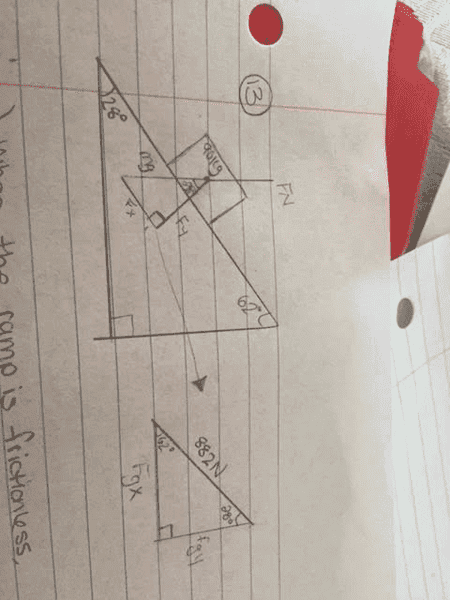# Box Pushed Up an Incline Ramp: Basic Newton's Law Questions

## Homework Statement

A 90 kg box is pushed by a horizontal force F at constant speed up a ramp inclined at 28°, as shown. Determine the magnitude of the applied force.
1. when the ramp is frictionless.
2. when the coefficient of kinetic friction is 0.18F=mg
FN=mg
μ= Fk/FN

## The Attempt at a Solution

a) mg=(90)((.8)= 882N=Fg

sin28=Fgx/882N
(0.469)(882)= Fgx
414N=Fgx= magnitude of applied force

b) FN=mg= 882N
μ=Fk/FN
0.18=Fk/882
(882)(0.18)= Fk
Fk= 158.76

** This is where I am unsure of my approach**

Fapplied= Fgx+Fk
=414N+158.76 N
= 572N

That seems like too much to me, but I'm not quite sure. Does it look like I have that equation correct ,or am I supposed to subtract the value I found for Fk from FgX, or something totally different. Any help would be very much appreciated

FN=mg= 882N
μ=Fk/FN
0.18=Fk/882
(882)(0.18)= Fk
Fk= 158.76

You have not drawn FN correctly . Redraw correctly and try again .

Hope this helps .

You have not drawn FN correctly . Redraw correctly and try again .

Hope this helps .
oh okay, is it opposing the Fy aspect instead of Fg? I was confused when I was drawing that! Thank you for your response

SammyS
Staff Emeritus